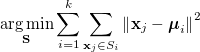# k-means clustering (naive)

k-means is a method to partition a set of n examples in k clusters Si in S around the nearest center in order to find the best assignment:The problem is complex, so the standard k-means algorithm is based on a two steps heuristics:

• Assignment step: assign each example at the cluster with the nearest center

• Update step: update the center computing the mean on the newly assigned examples

The algorithm converges when the assignments no longer change.

The main loop of the algorithm is:

To test this naive implementation we can run it on the famous Iris dataset. In this demo we shuffle the dataset with a fixed seed for reproducibility reasons: